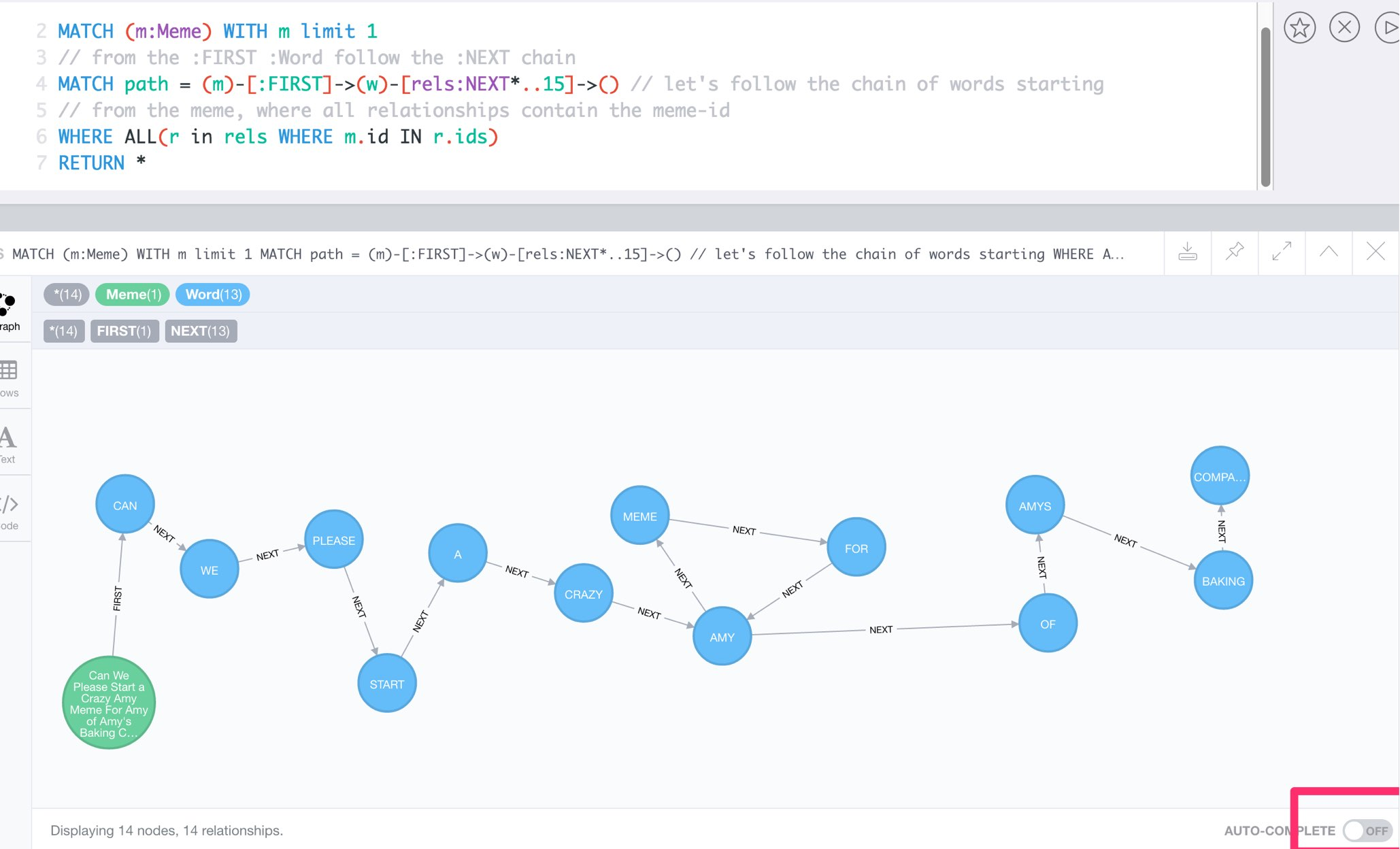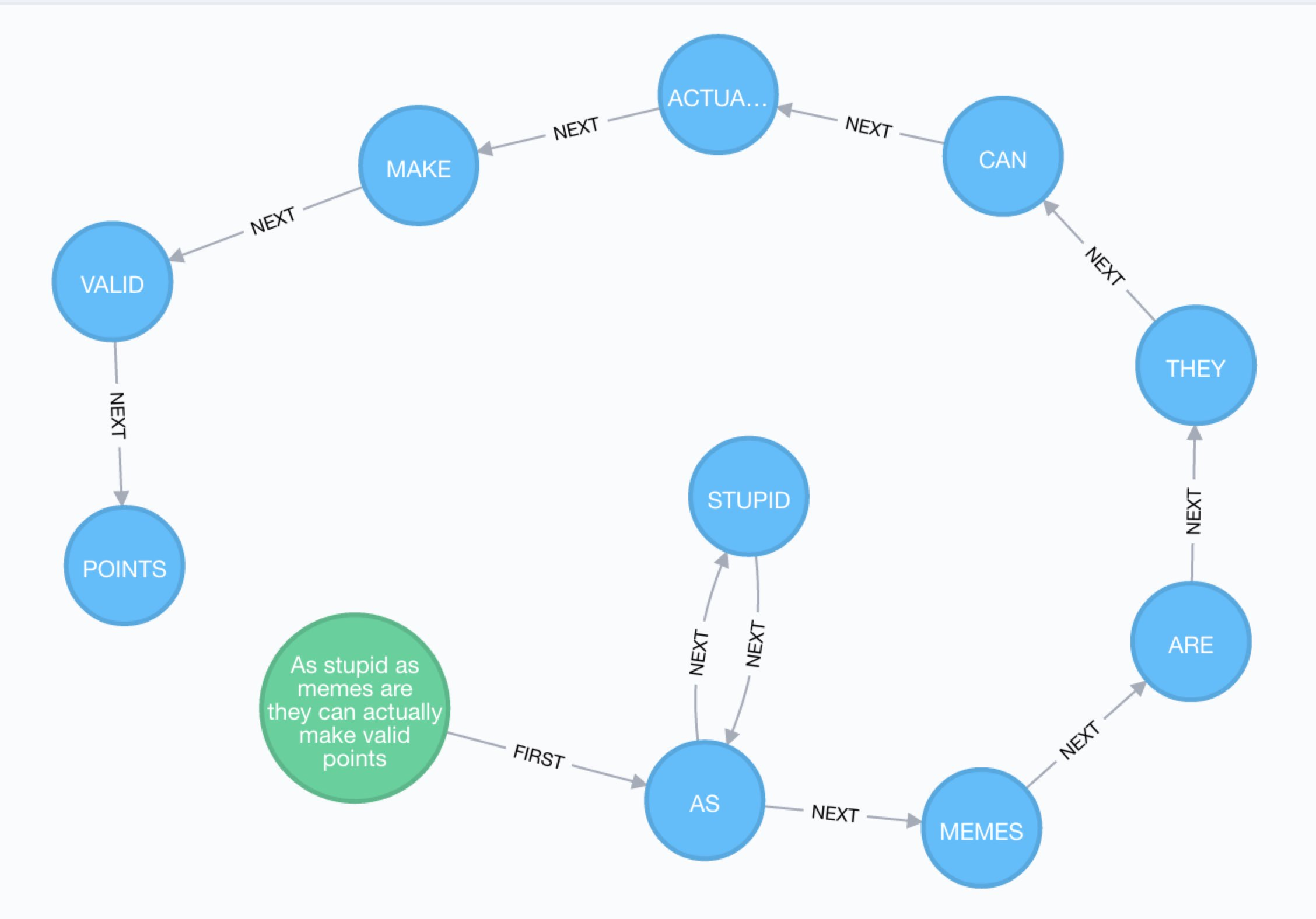Saturday night after not enough drinks, I came across these tweets by @LeFloatingGhost.

They definitely look like a meme graph.

We can do that too

../img/neo4j-memes.gif[Animated GIF (15M)]

## Find us some memes

There is this really nice CSV from Reddit of the top memes around:

And use an empty Neo4j Sandbox from http://neo4jsandbox.com.

## What’s the data?

### Check CSV

``````WITH 'https://raw.githubusercontent.com/umbrae/reddit-top-2.5-million/master/data/memes.csv' as url
RETURN count(*);``````
``````WITH 'https://raw.githubusercontent.com/umbrae/reddit-top-2.5-million/master/data/memes.csv' as url
RETURN row limit 10;``````

``````WITH 'https://raw.githubusercontent.com/umbrae/reddit-top-2.5-million/master/data/memes.csv' as url
WITH row LIMIT 100
CREATE (m:Meme) SET m=row // we take it all into Meme nodes``````

### Get some memes

``MATCH (m:Meme) return m limit 25;``
``MATCH (m:Meme) return m.id, m.title limit 5;``

## But we want the words !

Let’s grab the first meme and get going.

### Split the text into words.

``````MATCH (m:Meme) WITH m limit 1
RETURN split(m.title, " ") as words;``````

### Shout it aloud

``````MATCH (m:Meme) WITH m limit 1
RETURN split(toUpper(m.title), " ") as words;``````

## Remove Punctuation

Create an array of punctuation with split on empty string.

``return split(",!?'.","");``

### And replace each of the characters with nothing ''

``return reduce(s="a?b.c,d", c IN split(",!?'.","") | replace(s,c,''));``

### We got us some nice words

``````MATCH (m:Meme)  WITH m limit 1
// lets split the text into words
RETURN split(reduce(s=toUpper(m.title), c IN split(",!?'.","") | replace(s,c,'')), " ") as words;``````

## Enough words, where are the nodes?

### Let’s create some word nodes

(merge does get-or-create)

``````MATCH (m:Meme)  WITH m limit 1
WITH split(reduce(s=toUpper(m.title), c IN split(",!?'.","") | replace(s,c,'')), " ") as words, m
MERGE (a:Word {text:words})
MERGE (b:Word {text:words});``````

### Our first two words

``MATCH (n:Word) RETURN n;``

### Unwind the ra(n)ge

But we want all in the array, so let’s unwind a range.

``````MATCH (m:Meme)  WITH m limit 1
WITH split(reduce(s=toUpper(m.title), c IN split(",!?'.","") | replace(s,c,'')), " ") as words, m

UNWIND range(0,size(words)-2) as idx // turn the range into rows of idx

MERGE (a:Word {text:words[idx]})
MERGE (b:Word {text:words[idx+1]});``````
``MATCH (n:Word) RETURN n;``

## No Limits

``````MATCH (m:Meme) WITH m // no limits
WITH split(reduce(s=toUpper(m.title), c IN split(",!?'.","") | replace(s,c,'')), " ") as words, m

UNWIND range(0,size(words)-2) as idx // turn the range into rows of idx

MERGE (a:Word {text:words[idx]})
MERGE (b:Word {text:words[idx+1]});``````
``MATCH (n:Word) RETURN count(*);``

## Chain up the memes

Connect the words via :NEXT and store the meme-ids on each rel in an `ids` property

And for the first word (idx = 0) let’s also connect the Meme node to the Word `a`

``````MATCH (m:Meme) WITH m
WITH split(reduce(s=toUpper(m.title), c IN split(",!?'.","") | replace(s,c,'')), " ") as words, m
UNWIND range(0,size(words)-2) as idx // turn the range into rows of idx
MERGE (a:Word {text:words[idx]})
MERGE (b:Word {text:words[idx+1]})

// Connect the words via :NEXT and store the meme-ids on each rel in an `ids` property
MERGE (a)-[rel:NEXT]->(b) SET rel.ids = coalesce(rel.ids,[]) + [m.id]

// to later recreate the meme along the next chain
// connect the first word to the meme itself
WITH * WHERE idx = 0
MERGE (m)-[:FIRST]->(a);``````

Yay done!

``````MATCH (n:Word)
RETURN n LIMIT 33;``````

## Which words appear most often

``````MATCH (w:Word)
WHERE length(w.text) > 4
RETURN w.text, size( (w)--() ) as relCount
ORDER BY relCount DESC LIMIT 10;``````
```╒══════════════════╤══════════╕
│"w"               │"relCount"│
╞══════════════════╪══════════╡
│{"text":"AFTER"}  │"56"      │
├──────────────────┼──────────┤
│{"text":"REDDIT"} │"34"      │
├──────────────────┼──────────┤
├──────────────────┼──────────┤
│{"text":"TODAY"}  │"33"      │
├──────────────────┼──────────┤
│{"text":"SCUMBAG"}│"32"      │
├──────────────────┼──────────┤
│{"text":"EVERY"}  │"31"      │
├──────────────────┼──────────┤
│{"text":"FIRST"}  │"30"      │
├──────────────────┼──────────┤
│{"text":"ALWAYS"} │"28"      │
├──────────────────┼──────────┤
│{"text":"FRIEND"} │"27"      │
├──────────────────┼──────────┤
│{"text":"THOUGHT"}│"24"      │
└──────────────────┴──────────┘```

## Now let’s find our memes again

``````// first meme
MATCH (m:Meme) WITH m limit 1
// from the :FIRST :Word follow the :NEXT chain
MATCH path = (m)-[:FIRST]->(w)-[rels:NEXT*..15]->() // let's follow the chain of words starting
// from the meme, where all relationships contain the meme-id
WHERE ALL(r in rels WHERE m.id IN r.ids)
RETURN *;``````### Show meme by id

We can also get meme from the CSV list, e.g. id '1kc9p2' - 'As stupid as memes are they can actually make valid points'

``````MATCH (m:Meme) WHERE m.id = '1kc9p2'

MATCH path = (m)-[:FIRST]->(w)-[rels:NEXT*..15]->()
WHERE ALL(r in rels WHERE m.id IN r.ids)

RETURN *;``````Done. Enjoy !